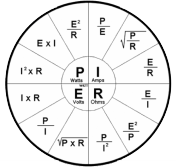Toll Free: 800.886.7001

# Engineering Resources

## Conversions and Definitions

1 Cubic Foot of Natural Gas = 1,000 BTU’s

1 Therm Natural Gas = 100,000 BTU’s

1 Unit Natural Gas (10 Therms) = 1,000,000 BTU’s

1 Cubic Foot Propane Gas = 2,500 BTU’s

1 Gallon #2 Oil = 140,000 BTU’s

1lb Steam = 1,000 BTU’s

1lb Water = 1lb Steam [weight]

1 Boiler HP = 34.5 lb/hr Steam = 33,479 BTUH Output = 0.069 GPM Water Evaporation Rate = 139 SQ FT EDR (Equivalent Direct Radiation)

1 Gallon of Water = 8.345 lbs = 7.48 cu ft

1 KWH Electric = 3,412 BTUH
Normal Stack Temp on a 15 PSI Steam Boiler is 375℉
Normal Stack Temp on a 150 PSI Steam Boiler is 475℉
1 PSI = 2.31 Ft of Head
1 Ft of Head = .433 PSI
28 Inches Water Column = 1 PSI

SATURATED STEAM TEMPERATURES
15 PSIG = 250℉
30 PSIG = 274℉
50 PSIG = 298℉
150 PSIG = 366℉
WATER SYSTEM EQUATION
BTUH = 500 x GPM x ΔT (℉)
or
GPM = BTUH / 500 x ΔT (℉)

VELOCITY OF WATER FLOWING IN A PIPE

V = GPM x 0.41 / d squared

V = ft / sec

d = ID in inches

PUMP HP = GPM x ft hd x specific gravity / 3960 x % eff or GPM x PSI x specific gravity / 1713 x % eff

Specific gravity water = 1VENTING CATEGORIES FOR GAS FIRED EQUIPMENT
Category I – Negative Pressure, Non-Condensing
Category II – Negative Pressure, Condensing
Category III – Positive Pressure, Non-Condensing
Category IV – Positive Pressure, Condensing

WATER
Water is alkaline at pH above 7.0
Water is neutral at pH of 7.0
Water is acid at pH below 7.0
1 Grain of hardness = 17.1 parts per million
CFM = BTUH / 1.08 x ΔT (℉)
BTUH = CFM x 1.08 x ΔT (℉)
1 Ton of Refrigeration = 12,000 BTUH
1 Cooling tower ton = 15,000 BTUH

day-trips